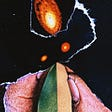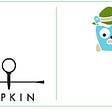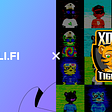# Using shaders to make trippy backgrounds

For my game EAT GIRL, I made a lot of wonky animated backgrounds to give the game a disorienting tone. Some people have asked me how I made the backgrounds, and the answer is shaders! Shaders can seem like black magic, and they kind of are, but they’re a surprisingly simple kind of magic that anybody can conjure.

This is a very non-technical overview of the “Wavy” shader, which is the shader I used for most of the level backgrounds in the game.

To demonstrate the shader, I will be using this simple background. It’s just circles!

# Using a pixel shader to distort an image

There’s quite a few different kinds of shaders, but to distort an image we’ll use a pixel shader.

A pixel shader is a small program that runs on the GPU and changes pixels before they’re drawn to the screen. Pixel shaders in LÖVE (the framework I used to make the game) take a few inputs — most important to us are the texture that we’re drawing and the coordinates of the pixel that we’re changing.

This is the default pixel shader LÖVE uses. It takes a texture, multiplies it by the global blend color, and outputs it.

`vec4 effect(vec4 color, Image tex, vec2 texture_coords, vec2 screen_coords){    vec4 texturecolor = Texel(tex, texture_coords);    return texturecolor * color;}`

We’re not so concerned with changing the colors of pixels — we want to move them around. We can do this by changing the `texture_coords`:

`vec4 effect(vec4 color, Image tex, vec2 texture_coords, vec2 screen_coords){    texture_coords.x += .5; // shift the pixels to the left    vec4 texturecolor = Texel(tex, texture_coords);    return texturecolor * color;}`

In this example, for each pixel in the original texture, we’re drawing the pixel half of the texture’s width to the right, effectively moving every pixel to the left.

You can also seem some weird stuff on the right side of the screen — that’s just the right edge of the texture getting repeated infinitely. We can alleviate this by using the modulo function to wrap the texture coordinates back around when they leave the 0–1 range.

`vec4 effect(vec4 color, Image tex, vec2 texture_coords, vec2 screen_coords){    texture_coords.x += .5; // shift the pixels to the left    texture_coords.x = mod(texture_coords.x, 1.);    vec4 texturecolor = Texel(tex, texture_coords);    return texturecolor * color;}`

That looks more sensible, but it’s boring! How can we distort the image in an interesting way?

This is where the sine function comes in. To avoid getting into too much math, it’s a function that oscillates back and forth as its input increases. So let’s use the sine function to shift the pixels back and forth as we move down the texture.

`vec4 effect(vec4 color, Image tex, vec2 texture_coords, vec2 screen_coords){    texture_coords.x += .5 * sin(texture_coords.y * 5.);    texture_coords.x = mod(texture_coords.x, 1.);    vec4 texturecolor = Texel(tex, texture_coords);    return texturecolor * color;}`

Wow, that’s wavy! But that’s kind of a lot. Let’s define some uniform variables so we can easily change parameters of the shader. In a game, you’d definitely take advantage of the fact that you can control the shader parameters from the Lua code, but for the sake of this article, we’ll just tweak the values in the shader code itself.

`uniform float amount = .1;uniform float density = 10.;vec4 effect(vec4 color, Image tex, vec2 texture_coords, vec2 screen_coords){    texture_coords.x += amount * sin(texture_coords.y * density);    texture_coords.x = mod(texture_coords.x, 1.);    vec4 texturecolor = Texel(tex, texture_coords);    return texturecolor * color;}`

Here I added parameters to control how wavy the image is and how vertically scrunched the waves are.

So this is a lot nicer, but it’s still very static looking. Can we add some more motion to this background? Of course! We can input more things into the `sin` function than just the y position of the pixel. Let’s add a uniform called `time`, and we’ll keep a time variable in our Lua code that steadily increases and send it to the shader. We’ll also add a speed uniform.

`uniform float amount = .1;uniform float density = 10.;uniform float speed = 1.;uniform float time = 0.;vec4 effect(vec4 color, Image tex, vec2 texture_coords, vec2 screen_coords){    texture_coords.x += amount * sin(texture_coords.y * density + speed * time);    texture_coords.x = mod(texture_coords.x, 1.);    vec4 texturecolor = Texel(tex, texture_coords);    return texturecolor * color;}`

Now we’re getting somewhere! But it’s not ✨trippy✨ enough. Let’s try some weird shit.

# Interlacing

This is one technique we can use that’s very Earthbound. For every other row of pixels, we’ll reverse the direction that we shift them.

`uniform float amount = .1;uniform float density = 10.;uniform float speed = 1.;uniform bool interlace = true;uniform float time = 0.;vec4 effect(vec4 color, Image tex, vec2 texture_coords, vec2 screen_coords){    // get the amount to shift this row of pixels    float offset = amount * sin(texture_coords.y * density + speed * time);    if (interlace && mod(screen_coords.y, 2.) > 1.) offset *= -1.;    texture_coords.x += offset;    texture_coords.x = mod(texture_coords.x, 1.);    vec4 texturecolor = Texel(tex, texture_coords);    return texturecolor * color;}`

# Tangent

We don’t have to use the sine function to modulate the horizontal shift amount of the pixels. Let’s try `tan`:

`uniform float amount = .1;uniform float density = 10.;uniform float speed = 1.;uniform bool interlace = true;uniform float time = 0.;vec4 effect(vec4 color, Image tex, vec2 texture_coords, vec2 screen_coords){    // get the amount to shift this row of pixels    float offset = amount * tan(texture_coords.y * density + speed * time);    if (interlace && mod(screen_coords.y, 2.) > 1.) offset *= -1.;    texture_coords.x += offset;    texture_coords.x = mod(texture_coords.x, 1.);    vec4 texturecolor = Texel(tex, texture_coords);    return texturecolor * color;}`

Weird!

# Square root

Let’s square root the offset just for fun.

`uniform float amount = .1;uniform float density = 10.;uniform float speed = 1.;uniform bool interlace = true;uniform float time = 0.;vec4 effect(vec4 color, Image tex, vec2 texture_coords, vec2 screen_coords){    // get the amount to shift this row of pixels    float offset = amount * sin(texture_coords.y * density + speed * time); // going back to sin for now    if (interlace && mod(screen_coords.y, 2.) > 1.) offset *= -1.;    offset = sqrt(offset);    texture_coords.x += offset;    texture_coords.x = mod(texture_coords.x, 1.);    vec4 texturecolor = Texel(tex, texture_coords);    return texturecolor * color;}`

What makes this effect janky is that sometimes our offset is negative, but the square root of a negative number is not a real number. Most programming languages would complain if we tried to do this, but GLSL just rolls with it.

It’s also more interesting to put the square root after the interlacing — that way, every other row of pixels responds differently to being square rooted.

# Vertical shifting

What if we use the offset to change the y position of a pixel instead of the x position?

`uniform float amount = .1;uniform float density = 10.;uniform float speed = 1.;uniform bool interlace = true;uniform float time = 0.;vec4 effect(vec4 color, Image tex, vec2 texture_coords, vec2 screen_coords){    // get the amount to shift this row of pixels    float offset = amount * sin(texture_coords.y * density + speed * time); // going back to sin for now    if (interlace && mod(screen_coords.y, 2.) > 1.) offset *= -1.;    offset = sqrt(offset);    texture_coords.y += offset;    texture_coords.y = mod(texture_coords.y, 1.);    vec4 texturecolor = Texel(tex, texture_coords);    return texturecolor * color;}`

Nice! That’s also very Earthbound.

I hope this gave you some useful, albeit non-technical insight into how you can use shaders to make some trippy distortion effects. Basically just experiment with weird math operations; you can’t go wrong!

If you want to see these kinds of effects in action, check out EAT GIRL! It’s \$5 on itch.io!

--

--

--

## More from tesselode

musician / game developer / software developer | tesselode.github.io

Love podcasts or audiobooks? Learn on the go with our new app.

## Let’s stack the ODROID-N2## CS373 Fall 2020: Michael Chan## ScholarX — A path towards personal and career development.## From Freelance to Full Stack & Hired — A Lambda School/Labs Experience## Distributed tracing setup in GKE — Jaeger / Zipkin — Google Cloud Platform## xDAI Tigers Integrate LI.FI’s Widget## NVIDIA DALI: Speeding up PyTorch## tesselode

musician / game developer / software developer | tesselode.github.io

## Flutter vs. Kotlin app development: Trends, statistics, and adoption